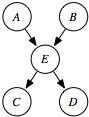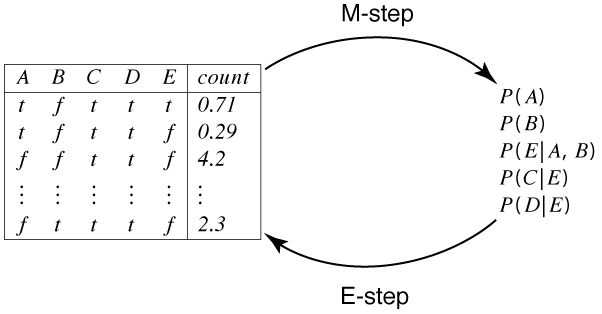### 11.2.2 Unobserved Variables

The next simplest case is one in which the model is given, but not all variables are observed. A hidden variable or a latent variable is a variable in the belief network models whose value is not observed. That is, there is no column in the data corresponding to that variable.

Model Data ProbabilitiesA B C D t f t t f t t t t t f t ···
 P(A) P(B) P(E|A,B) P(C|E) P(D|E)
Figure 11.6: Deriving probabilities with missing data

Example 11.5: Figure 11.6 shows a typical case. Assume that all of the variables are binary. The model contains a variable E that is not in the database. The data set does not contain the variable E, but the model does. We want to learn the parameters of the model that includes the hidden variable E. There are 10 parameters to learn.

Note that, if E were missing from the model, the algorithm would have to learn P(A), P(B), P(C|AB), P(D|ABC), which has 14 parameters. The reason to introduce hidden variables is to make the model simpler and, therefore, less prone to overfitting.

The EM algorithm for learning belief networks with hidden variables is essentially the same as the EM algorithm for clustering. The E step can involve more complex probabilistic inference as, for each example, it infers the probability of the hidden variable(s) given the observed variables for that example. The M step of inferring the probabilities of the model from the augmented data is the same as the fully observable case discussed in the previous section, but, in the augmented data, the counts are not necessarily integers.Figure 11.7: EM algorithm for belief networks with hidden variables